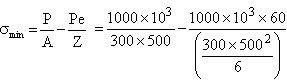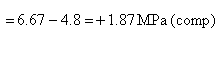Courses

# Test: Environmental Engineering & RCC- 1

## 25 Questions MCQ Test GATE Civil Engineering (CE) 2022 Mock Test Series | Test: Environmental Engineering & RCC- 1

Description
This mock test of Test: Environmental Engineering & RCC- 1 for GATE helps you for every GATE entrance exam. This contains 25 Multiple Choice Questions for GATE Test: Environmental Engineering & RCC- 1 (mcq) to study with solutions a complete question bank. The solved questions answers in this Test: Environmental Engineering & RCC- 1 quiz give you a good mix of easy questions and tough questions. GATE students definitely take this Test: Environmental Engineering & RCC- 1 exercise for a better result in the exam. You can find other Test: Environmental Engineering & RCC- 1 extra questions, long questions & short questions for GATE on EduRev as well by searching above.
QUESTION: 1

Solution:
QUESTION: 2

Solution:
QUESTION: 3

### Tensile strength of concrete is measured by

Solution:
QUESTION: 4

The critical section for beam shear when support is under tension is (d = effective depth)

Solution:
QUESTION: 5

Assertion (A): Minimum shear reinforcement using stirrups must be provided even if TV < 0.5 Tc
Reason (R): In above statement minimum shear reinforcement is to take care of sudden accidental increase of shear force in rear occasions

Solution:
QUESTION: 6

Which of the following statements are true

P. Bond is based on stress compatability
Q. limit state method is strain oriented approach
R. Working stress method of design is theoretical approach

Solution:
QUESTION: 7

Anchorage value of a hook in RCC is

Solution:
*Answer can only contain numeric values
QUESTION: 8

Modulus of elasticity of M35 concrete is _____ Mpa

Solution: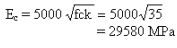QUESTION: 9

Axial load carrying capacity of a column of given material length cross sectional area depends on

Solution:
QUESTION: 10

Maximum upward deflection of prestressed concrete member is limited to

Solution:
QUESTION: 11

The slope of a 1.0 m diameter concrete sewer laid at a slope of 1 in 1000, develops a velocity of 1 m/s, when flowing full. When it is flowing half-full, the velocity of flow through the sewer will be

Solution: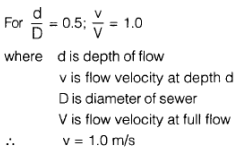QUESTION: 12

Which one of the following pollutants or pairs of pollutants is formed due to photochemical reactions?

Solution:

Photochemical reactions form O3, PAN, formaldehyde, etc.

QUESTION: 13

AT- beam roof section has following details.
Thickness of slab = 100 mm
Width of rib = 230 mrn
Overall depth of beam = 350 mm
Centre to centre distance of beams = 4m
Effective simply supported span of beams = 6m
Effective flange width is

Solution: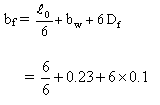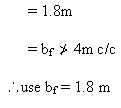QUESTION: 14

A doubly reinforced beam of width 350 mm and effective depth 900 mm, with cover to compression steel is 50 mm. Reinforcement in tension zone is 5 bars of 20 mm dia and 2 bars of 20 mm dia in compression

M20 concrete are used

The depth of Neutral axis, if compressive strain in compression steel is 0.0028, is

Solution: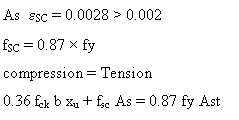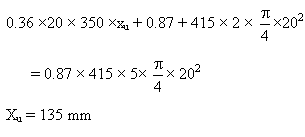QUESTION: 15

In limit state design method

P. Tensile strength of concrete is ignored
Q. Plane sections may not remain plane after bending
R. Maximum strain in concrete is 0.0035

Which of the following statements are true

Solution:
QUESTION: 16

Match the following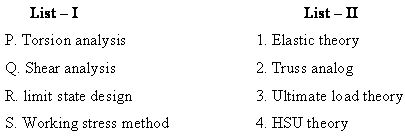Solution:
QUESTION: 17

A structure at working condition the following loads are existing.
DL = 40 kN/m;
LL= 60 kN/m;
WL = 30 kN/m;
EQ = 35 kN/m

The factored load for limit state of collapse is

Solution:

1. 1.5 (LL) + 1.5 (DL) = 150 kN/m
2. 1.5 (DL) + 1.5 (EQ) = 112.5 kN/m
3. 1.2 (LL) + 1.2 (DL) + 1.2(EQ) = 162.kN/m

QUESTION: 18

A rectangular column 600 x 450 mm is having un supported length of 4m, both ends arerestrained against rotation and held in position. The column should be designed as

Solution: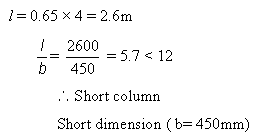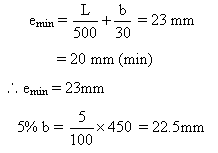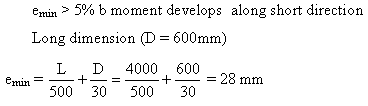Wo moment develops along longer direction
∴ The column must be designed as short axially loaded with uni-axial BM

QUESTION: 19

A rectangular beam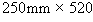mm overall is reinforced with 4 bars of 20 mm diameter in tension zone. Assume both tension and compression steels are yielded. M20 concrete and Fe 415 steel are used. Effective corer is 40mm, for both tension and compression steels.

Q.

The beam should be

Solution:
QUESTION: 20

A rectangular beam 250mm x 520 mm overall is reinforced with 4 bars of 20 mm diameter in tension zone. Assume both tension and compression steels are yielded. M20 concrete and Fe 415 steel are used. Effective corer is 40mm, for both tension and compression steels.

Q.

Limiting moment of resistance of the beam is

Solution: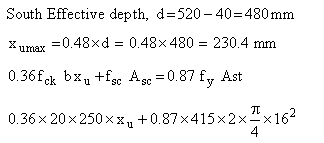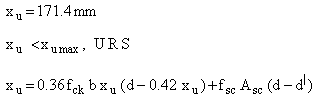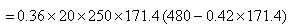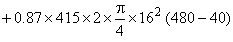= 190kN-m

QUESTION: 21

A rectangular beam of rectangular cross-section 200 mm x 450 mm (effective) is subjected to a factored shear force of 250 kN. The maximum shear resistance of the beam Tcmax = 2.5 MPa. The beam is

Solution: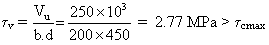Not safe in shear. It must be redesigned or made safe by reducing factored shear force to = Tvb.d = 225 kN

*Answer can only contain numeric values
QUESTION: 22

Limiting area of steel for a rectangular beam of 250 rnrn wide and 450 rnm effective depth with M20 concrete and Fe 415 steel is ________ mm2

Solution: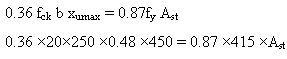*Answer can only contain numeric values
QUESTION: 23

An isolated T-bearn has effective simply supported span of 10 m. Actual width of flange is 2 m, width of beam 250 mm effective flange width is _______ m

Solution: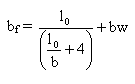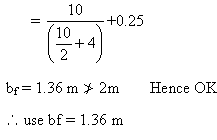*Answer can only contain numeric values
QUESTION: 24

In a PSC beam shown in figure having parabolic cable with an effective preste res sing force of 2000 kN. The beam supports a udl of 20kN/m over a span of 8 m. The eccentricity required at the centre of bean is (e) ______ mm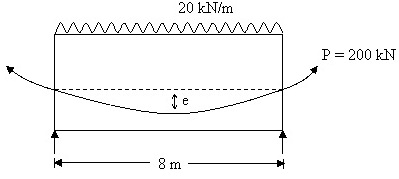Solution: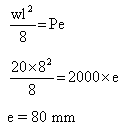QUESTION: 25

A prestressed concrete section 300 trim x 500 mm is prestressed by a straight cable at 60mm eccentricity. Prstressed force in the cable is 1000 kN. The minimum prestress developed only due to pre stresing force is

Solution: